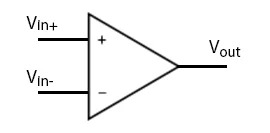﻿

# Op amp

## What is an Op amp?

### Definition

Operational amplifier: The ideal op amp is an amplifier with infinite input impedance, infinite open-loop gain, zero output impedance, infinite bandwidth, and zero noise. It has positive and negative inputs which allow circuits that use feedback to achieve a wide range of functions.Using op amps, it's easy to make amplifiers, comparators, log amps, filters, oscillators, data converters, level translators, references, and more. Mathematical functions like addition, subtraction, multiplication, and integration can be easily accomplished.

Practical, real-world op amps have finite characteristics but in most applications, are close enough to the ideal to make a huge range of inexpensive, high-performance analog applications possible. They are the building block for analog design.

One key to op amp design is nodal analysis. Since the input impedance is infinite, the current in and out of the + and − input nodes defines the circuit's behavior.

### Synonyms

opamp
Find a term alphabetically: